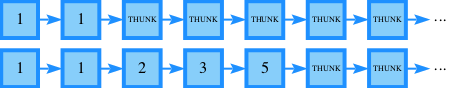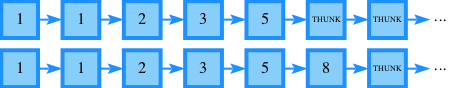## Thursday, June 18, 2015

### Will it memoize?

In this post, I will try several variants of a simple memoizing Haskell program and test whether the program actually memoizes or not. This will give us a better grasp on sharing.
##### Fibonacci
If you've been studying Haskell for any length of time, you have probably already stumbled upon the following unusual definition of the Fibonacci sequence.
``````fibs = 1 : 1 : zipWith (+) fibs (tail fibs)
``````
If I print the fourth element of this list, how many additions will be evaluated? What if I then print the fifth element? Would you believe me if I told you that the answer depends on whether the `NoMonomorphismRestriction` extension is enabled or not? The answer also depends on various other details, which we will explore in this post.
In order to observe the additions as they are being evaluated, let's create a variant which prints a message to the console when it is called.
``````import Debug.Trace

fibs = 1 : 1 : zipWith noisyAdd fibs (tail fibs)
fib n = fibs !! n
``````
So, how many additions when I print the fourth element?
``````> fib 4
5
``````
Three, because that's the number of entries in `[2..4]`, and at each of those indices a single addition is performed to compute the next number out of the two previous numbers.How many additions when I then print the fifth?
``````> fib 5
8
``````
Only one! There are four entries in `[2..5]`, but entries 2 through 4 have already been evaluated during our previous query. Implementing `fib` via a list of all possible results has effectively memoized our function.##### Inside a `where` clause
The fact that `fib` is using a list for memoization purposes is an implementation detail. Let's hide the list inside the function's `where` clause.
``````fib n = fibs !! n
where
fibs = 1 : 1 : zipWith noisyAdd fibs (tail fibs)
``````
Will it memoize?
``````> fib 4
5
> fib 5
8
``````
It did not! The definitions in the `where` clause are local to the body of the function, which itself only exists once the argument `n` has been given. For this reason, each time `fib` is called, a new copy of `fibs` is allocated and the previously-memoized values are forgotten.Consider the following variant:
``````fib = \n -> fibs !! n
where
fibs = 1 : 1 : zipWith noisyAdd fibs (tail fibs)
``````
Will it memoize?
``````> fib 4
5
> fib 5
8
``````
It did! This time, the `where` block does not have access to the `n` argument, so it does not need to be instantiated on every call. As a result, all the calls to `fib` are backed by the same list, and memoization succeeds.##### NoMonomorphismRestriction
Let's enable the `NoMonomorphismRestriction` extension.
``````{-# LANGUAGE NoMonomorphismRestriction #-}

fib = \n -> fibs !! n
where
fibs = 1 : 1 : zipWith noisyAdd fibs (tail fibs)
``````
Adding GHC extensions allows extra non-standard features, but our existing standards-conforming code should work the same as before, shouldn't it? In particular, it should still memoize, right?
``````> fib 4
5
*Main> fib 5
8
``````
It did not! Enabling `NoMonomorphismRestriction` changed the type which GHC inferred for our definitions. With the extension turned on, GHC infers polymorphic types:
``````{-# LANGUAGE ScopedTypeVariables #-}

fib :: forall a. Num a => Int -> a
fib = \n -> fibs !! n
where
fibs :: [a]
fibs = 1 : 1 : zipWith noisyAdd fibs (tail fibs)
``````
The `Num a` constraint is implemented via an implicit dictionary argument, which leads to the same non-memoizing behavior we had earlier when the `n` argument was in scope inside the `where` block. Here, `Num a` is clearly in scope as well, otherwise `fibs` would not be able to add its elements together.Without `NoMonomorphismRestriction`, GHC infers monomorphic types:
``````fib :: Int -> Integer
fib = \n -> fibs !! n
where
fibs :: [Integer]
fibs = 1 : 1 : zipWith noisyAdd fibs (tail fibs)
``````
This time the global `Num Integer` instance is used, not an abstract `Num a` which could change from call to call. This allows the same `fibs` to be used on each call, which in turn allows the memoized values to be retained from call to call.##### Universal quantifiers

In the type signature `fib :: forall a. Num a => Int -> a`, there are three arguments of different kinds: the `a` is an implicit type argument, the `Num a` is an implicit dictionary argument, and the `Int` is an ordinary argument. We have seen that dictionary arguments and ordinary arguments both introduce a new scope and thereby hinder memoization. Is it also the case for type arguments?

To figure out the answer, let's construct a variant of `fib` which always adds integers and therefore doesn't need a dictionary argument, and also carries around a spurious polymorphic value on which no additions are performed. This way, we'll have a type argument but no dictionary argument.

``````fib :: forall a. Int -> a -> (Integer, a)
fib = pairWithFib
where
pairWithFib :: Int -> a -> (Integer, a)
pairWithFib n x = (fibs !! n, x)

fibs :: [Integer]
fibs = 1 : 1 : zipWith noisyAdd fibs (tail fibs)
``````
Will it memoize?
``````> fib 4 "foo"
(5,"foo")
*Main> fib 5 [42.0]
(8,[42.0])
``````

It did! Types get erased, they do not exist at runtime. In particular, instantiating `a` to `String` and later to `[Double]` does not involve passing around some runtime representation of `String` or `[Double]`, and it does not create a new copy of the `where` block.

It's a bit surprising, because this is a situation in which the evaluation semantics do not match up with the typing semantics. According to the type system, the `pairWithFib` in which `a` has been instantiated to `String` is not the same `pairWithFib` as the one in which `a` has been instantiated to `[Double]`, as those two `pairWithFib` instances have different types and thus cannot be interchanged. Yet at runtime, those two instances of `pairWithFib` are represented by the same identical value. The trick is that in the absence of any type class constraint, `pairWithFib` has no way to distinguish between the different types it is supposed to be instantiated at, so it is allowed to behave the same in all cases. In particular, it is allowed to be the same identical value in all cases.

##### Sharing

In the previous paragraph, I was careful to use the phrase "same identical value" as opposed to simply "the same value". By "identical", I mean that the two values have the same identity, which in turn means that they occupy the same space in memory.

In object-oriented languages, the concept of identity is very important. Suppose you hold references to two objects which happen to be equal to each other, in the sense that their corresponding fields are pairwise equal. Despite the fact that the objects are ostensibly equal, which of those two references you pass to a third party method matters because that third party might mutate one of the object's fields. If that happens, all the other references to this same identity, throughout your program, will also see the mutated field, while the references to other identities will not. And if you had passed the other reference, a different set of references, those which point to the other identity, will see their fields mutated. It's just normal pointer indirection, but it's something you always have to keep in mind in those languages, in order to avoid a family of problems known as aliasing issues.

In Haskell's immutable fragment, there are no aliasing issues, in the sense that calling a pure function on equal values will always yield equal results, regardless of the identity of those values. Nevertheless, the identity of those values does have its importance: identities do not impact correctness, but they do impact performance. Under some circumstances, excessive sharing can lead to poor performance, whereas in the case of memoization, sharing can increase performance by minimizing duplicated work.

The general rule explaining our results in all the previous sections is that memoization only happens if the data structure which holds the memoized values is shared between calls. To demonstrate this, our last example plays with sharing by specifying which copy of the `fibs` data structure is used to memoize which copy of the `fib` function.

``````mkFibs :: forall a. Num a => [a]
mkFibs = fibs
where
fibs :: [a]
fibs = 1 : 1 : zipWith noisyAdd fibs (tail fibs)

mkFib :: forall a. Num a => [a] -> Int -> a
mkFib = (!!)
``````
Let's begin by constructing two copies of the `fibs` data structure:
``````fibsA :: [Integer]
fibsA = mkFibs

fibsB :: [Integer]
fibsB = mkFibs
``````
And then two `fib` functions for each of the two copies of `fibs`:
``````fibA1 :: Int -> Integer
fibA1 = mkFib fibsA

fibA2 :: Int -> Integer
fibA2 = mkFib fibsA

fibB1 :: Int -> Integer
fibB1 = mkFib fibsB

fibB2 :: Int -> Integer
fibB2 = mkFib fibsB
``````
"Will it memoize" is now the wrong question to ask. Each function memoizes its own results, but which functions share their memoized results with each other?
``````> fibA1 4
5
> fibA2 5
8
> fibB1 4
5
> fibB2 5
As expected, functions which were created from the same copy of `fibs` share their results with each other.
2015-06-20 update: as /u/pycube correctly points out, the above technique only works when you want to make sure that something is shared. Preventing sharing is much harder, because optimizations tend to increase sharing. With `-O2`, for example, all the examples in this post memoize.